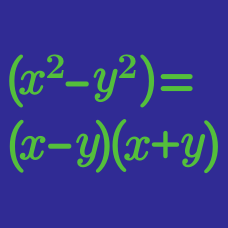Algebra

# Polynomial Factoring: Level 3 Challenges

The function $f(x) = x^5 + 6x^4 - 18x^3 - 10x^2 + 45x - 24$ has only four distinct roots, each of which is real. Let the four roots be $\alpha,\ \beta,\ \gamma,$ and $\delta,$ in no particular order. Also let $f'(x)$ denote the first derivative of $f(x).$ Evaluate $f'(\alpha)\times f'(\beta)\times f'(\gamma)\times f'(\delta).$

The function $f(x)=x^4+ax^3+bx^2+cx+d$ has four positive roots. We are also given that $(b-a)(a+b)+(d-c)(c+d)=2(ac-bd).$

What is the value of $(a+b+c+d)^2?$

If $a, b, c$ are real numbers such that $a^2 + b^2 +2c^2 - 4a+2c -2bc +5 = 0 ,$ then what is the value of $a+b-c$?

Let $f(x) = x^3 + 2x^2 + 3x + 2$ and $g(x)$ be a polynomial with integer coefficients. When $f(x)$ is divided by $g(x)$, it leaves quotient $q(x)$ and remainder $r(x)$, both of which have integer coefficients.

If $q(x) = r(x) \neq 1$ ,then find $g(5)$.

$\large x^6 + x^5 + 2x^4 + 2x^3 + 3x^2 + 3x + 3$

How many real roots does the polynomial above have?

×